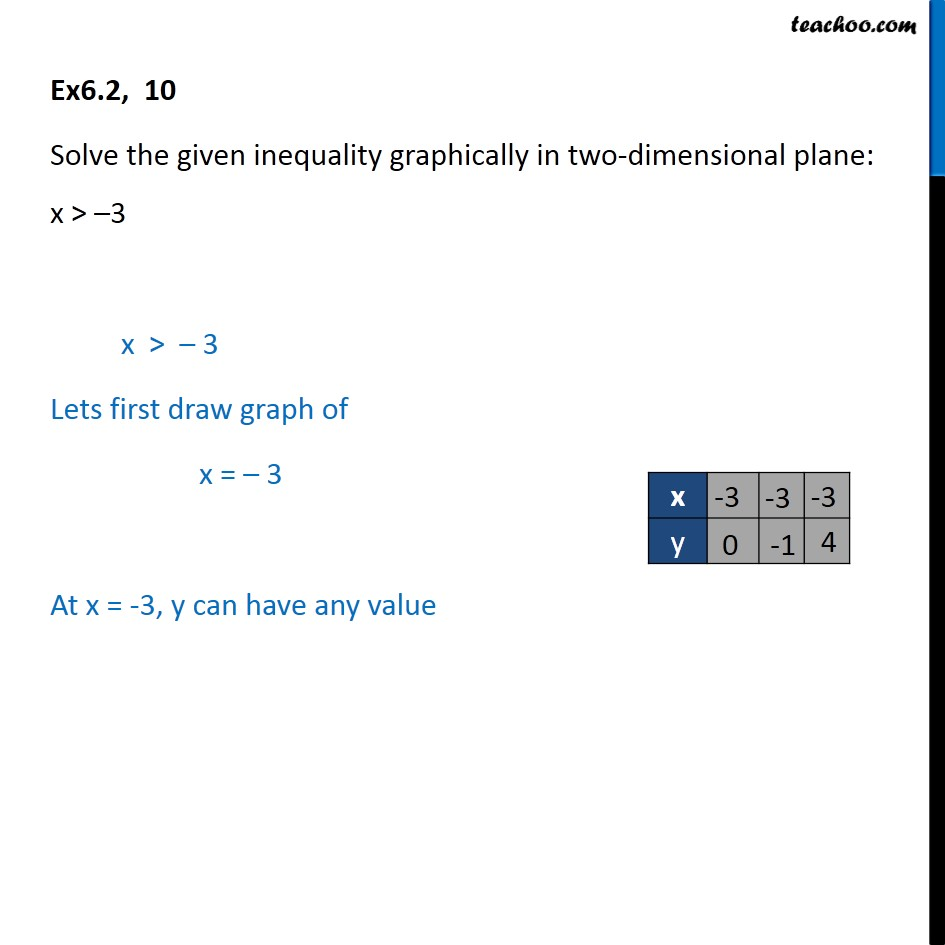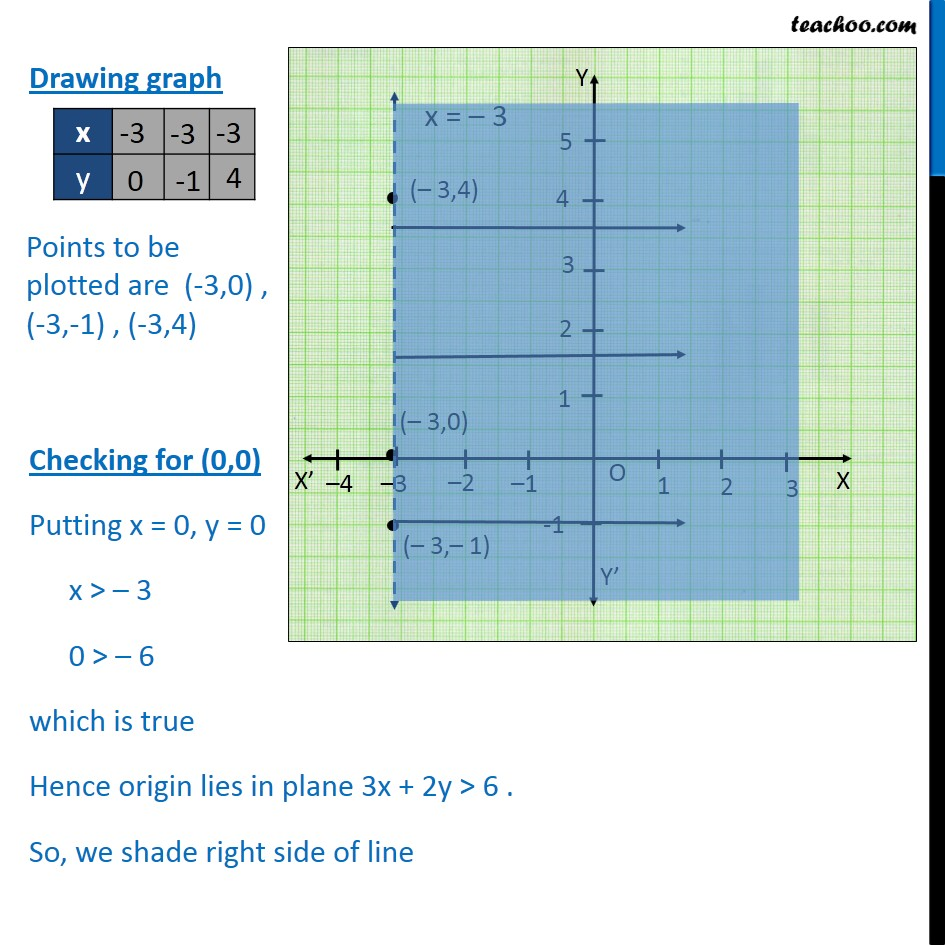Solving Linear Inequality Graphically

Chapter 5 Class 11 Linear Inequalities
Serial order wiseLearn in your speed, with individual attention - Teachoo Maths 1-on-1 Class

### Transcript

Ex6.2, 10 Solve the given inequality graphically in two-dimensional plane: x > –3 x > – 3 Lets first draw graph of x = – 3 At x = -3, y can have any value Drawing graph Checking for (0,0) Putting x = 0, y = 0 x > – 3 0 > – 6 which is true Hence origin lies in plane 3x + 2y > 6 . So, we shade right side of line